#### Chapter 7 Introduction to Euclid's Geometry R.D. Sharma Solutions for Class 9th Math VSAQ's

1. How many least number of distinct points determine a unique line?

Solution

If we have a single point A then we can draw infinite number of lines through it, while if we have two points A and B; then only one unique line passes through both of them.
When we have one point,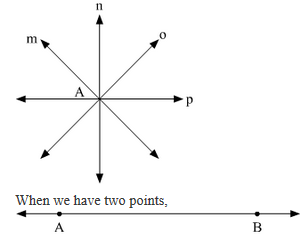Therefore, a minimum of two distinct points are required to determine a unique line.

2. How many lines can be drawn through both of the given points?
Solution
Given two distinct points, only one unique line can be drawn passing through both of them.
For example, if we have two distinct points A and B, only one line is there which passes through both of them .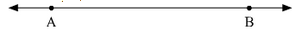3. How many lines can be drawn through a given point.
Solution

Given a single point, then we can draw infinite number of lines through that point.
For example: If we have a point A, then there are infinite numbers of lines passing through it.Here, lines m, n, o and p all pass through point A.

4. In how many points two distinct lines can intersect ?
Solution

Two distinct lines can intersect at only point, as there is only one common point between two intersecting lines.
For example, if l and m are two intersecting lines then there is only one common point O between them. This is the point of intersection.5. In how many points a line, not in a plane, can intersect the plane?
Solution
A line which is not present in the plane can intersect the plane at only one single point.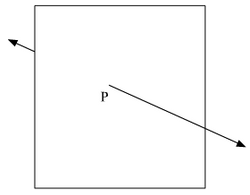6. In how many points two distinct planes can intersect?
Solution

Two distinct planes can intersect at only one unique line, which is common to both of them and one line is made up of infinite points.
Therefore, two distinct planes can intersect at infinite points.

7. In how many lines two distinct planes can intersect?
Solution

If we look at two intersecting plane, we can see that there is only one unique line at which the two planes intersect.Therefore, two distinct planes can intersect each other at a single unique line as only a single line is common between two intersecting planes.

8. How many least number of distinct points determine a unique plane?
Solution

If we have two distinct points, then we can draw infinite number of planes passing through those two points. While if we have three distinct non collinear points, only a single unique plane can be drawn passing through those three points.
Therefore, a minimum of three distinct non collinear points are required to get a unique plane.

9. Given three distinct points in a plane, how many lines can be drawn by joining them?
Solution
Given three distinct points A, B and C in a plane, they can either be collinear or non collinear.If they are collinear, then there can be only one line joining them.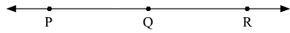If they are non collinear, then there can be three lines joining them.
For example, if we have three distinct non collinear points P, Q and R. Then we can draw three lines l, m and n joining them.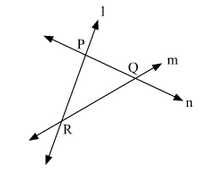10. How many planes can be made to pass through a line and a point not on the line?
Solution

Given a line and a distinct point not lying on the line, only a single plane can be drawn through both of them as there can be only plane which can accommodate both the line and the point together.
Let us take a line l and a point A, as we can see there can be only plane which pass through both of them.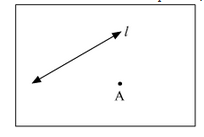11. How many planes can be made to pass through two points?
Solution

Given two distinct points, we can draw many planes passing through them. Therefore, infinite number of planes can be drawn passing through two distinct points or two points can be common to infinite number of planes.

12. How many planes can be made to pass through three distinct points?
Solution

The number of planes that can pass through three distinct points is dependent on the arrangement of the points.
a. If the points are collinear, then infinite number of planes may pass through the three distinct points.

b. If the points are non collinear, then only one unique plane can pass through the three distinct points.